# Explainable Forecasting and Nowcasting with State-of-the-art Deep Neural Networks and Dynamic Factor Model

Review this detailed tutorial with code and revisit the decades-long old problem using a democratized and interpretable AI framework of how precisely can we anticipate the future and understand its causal factors?

By Ajay Arunachalam, ML Specialist and Cloud Solution Architect | Senior Data Scientist.Image by author: Intuitive representation of model explainability & deep forecasting with DeepXF.

Through this post, we will go through one of the key evergreen business problems — i.e., Forecasting. We will see some quick theoretical insights about forecasting in general, followed by a step-by-step tutorial with multiple hands-on demo examples. We will see how the python library deep-xf is helpful to get started with building deep models at ease. Further, we will see how the model bias can also be estimated to keep track of the prediction bias & deviation. Also, we will see the explainability module part of this library, which can be used to understand model inferences and interpret results in a simple human-understandable form. For a quick introduction and all the use-case applications that could be supported by the deep-xf package, in general, is listed in the blog post here. Also, check out my other closely related detailed blog post on Nowcasting from here.

### Forecasting Problem Revisited

With the availability of low-cost hardware (sensors), Internet of Things (IoT), and Cloud architectures, data acquisition, data transfer, and data storage are no longer critical issues. Nowadays, we come across a bunch of streaming and wearable devices measuring several parameters round-the-clock at ease. In the past, time-series forecasting has been well-interpreted by linear, boosting, ensemble, and statistical methods. With the advancement in deep learning algorithms, certainly one can leverage the full potential benefits of it, as by far, we have seen the tremendous possibilities associated with deep learning techniques. We all know about several use cases with context to time-series streams where deep learning has been very pivotal. Let’s first understand the meaning of forecast in plain language, followed by the forecasting problem formulation in context to machine learning. “A forecast is a prediction or an educated good guess towards the future. Simply, it is like saying what is likely to happen in the future”. The diagram below presents a very basic forecasting model structure with input and output notations. Further, we see how a forecasting problem can be formulated given the historical time-step input sequences.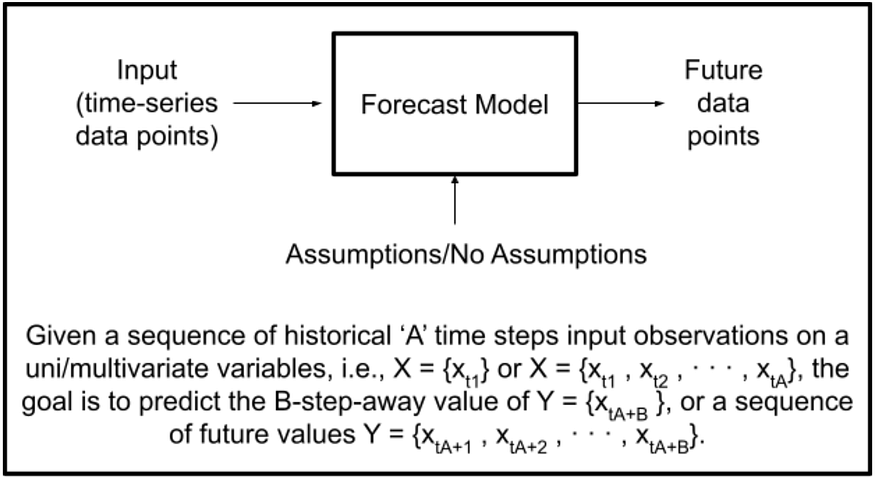Image by author: Simple forecast structure and forecasting problem formulation.

For a more intuitive, detailed explanation of time-series data, check here. And, for unsupervised feature selection from time-series data, check here.

### Conventional methods/techniques used for forecasting

Qualitative forecasting is based on pure opinion, intuition, and assumptions. While Quantitative forecasting uses statistical, mathematical, and machine learning models to make forecasts based on historical data.

From context to financial modeling, the top simple preferred methods include the ones shown in the table below (but not limited to these).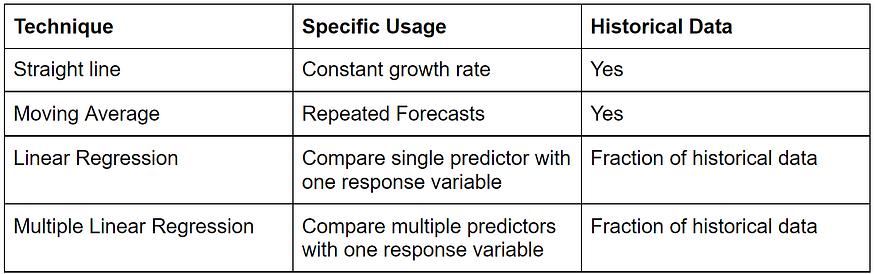Image by author: Basic types of forecasting methods to predict future values.

I will not dwell too much on the theoretical concepts of forecasting as that is not at all the focus of this blog post, and also, I won’t talk anything here about the different state-of-the-art deep learning network architectures as there are tons of good useful materials already available out there. So, let’s move on to the demo directly.

### Getting Started

We will see how to build a deep forecasting model with the Deep-XF library at ease. For installation, follow the given steps here, and for any other manual prerequisite installations, check here. We start by importing the library, as shown in the steps below. Next, we set custom pre-configurations for our deep learning-based forecast pipeline setting the parameters with set_model_config() function by choosing the appropriate user-specified algorithms (i.e., RNN, LSTM, GRU, BIRNN, BIGRU, and the input scaler, e.g., MinMaxScaler, StandardScaler, MaxAbsScaler, RobustScaler), and also using the past single period window as input, to forecast future values in sequence. Next, the set_variables() and get_variables() functions are used for setting and parsing the timestamp index, specifying the forecasting outcome variable of interest, sorting the data, removing duplicates, etc. Further, we can also select custom hyperparameters for training the forecast model with hyperparameter_config() function taking a bunch of key hyperparameters from learning ratedropoutweight decaybatch sizeepochs, etc.

Importing Deep-XF library

Custom user-specific pre-configurations

Setting Custom Model Hyperparameters

### Exploring and Preparing Data

Once all the configurations are set, we import the data, perform exploratory data analysis, preprocess the data, train the deep model, evaluate the model performance with bunch of key important metrics, followed by saving & outputting the forecast results to the disk, and also plotting them as interactive plotly plot of the forecast results for the user provided forecast window. Next, we estimate the forecast bias to validate if our model isn’t ending up over/under forecasting. And, finally, the explainability module helps us understand/interpret the model results of the inferred forecast for the user-inputted forecast sample.

We will see two different use cases here. The first one is using Alibaba cloud virtual machine’s data which is a multivariate dataset that is of seconds frequency interval (i.e., every 10 sec). The raw dataset is available from here. In contrast, the second one is the Sales & Marketing data which is also a multivariate dataset that is of monthly frequency interval. The raw dataset can be fetched from here.

Seconds Frequency Data Example —

Let’s first peek into the data by displaying a few rows. As seen, we have 6 columns, including the timestamp index.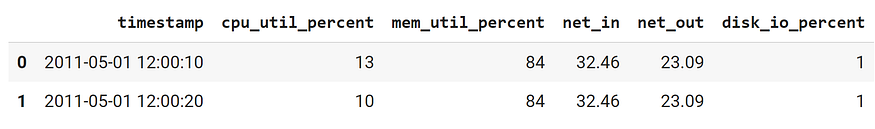Image by author: displaying first few rows from Alibaba cloud virtual machine’s dataset.

Next, let’s get the datatype information using the simple pandas info() function,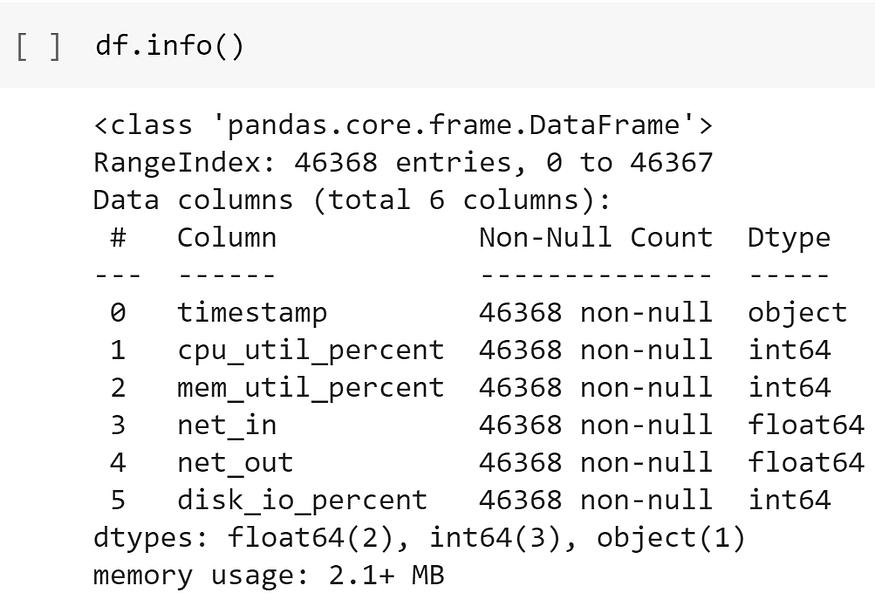Image by author: get columns information.

Next, we use the intuitive plot_dataset() function to visualize the trend of the outcome column wrt time index with the help of plotly interactive graph plots as shown below.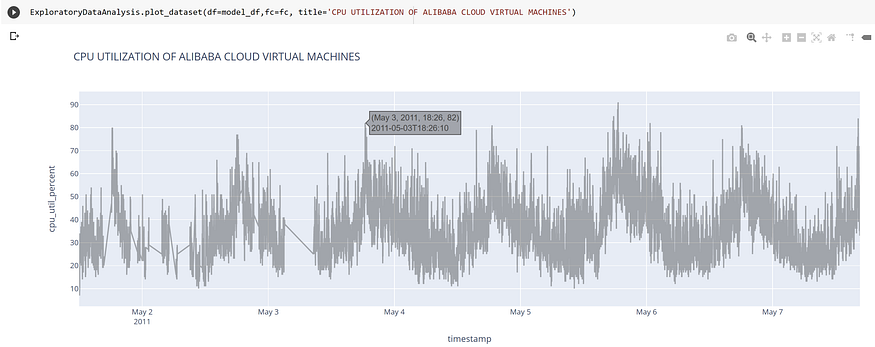Image by author: Interactive Trend Visualization Plot.

Further, we continue by doing Feature engineering. Feature Engineering is one of the key components of the machine learning lifecycle. In simple terms, it refers to the process of using domain knowledge to select, transform the most relevant variables, and along the process, derive new features from raw data. We start by generating simple features from timestamps using the generate_date_time_features_hour() function, followed by generating cyclic features from date, time using the generate_cyclic_features() method, and also generating other features relevant to the context of time-series data using the generate_other_related_features() method. Here, one can also use lagged observations as features. Further, one can also derive a bunch of several other useful features involving trend of values across various aggregation windows: i.e., change and rate of change in average, standard deviation across a certain window, the ratio of change, growth rate with standard deviation, change over time, rate of change over time, growth or decay, rate of growth or decay, count of values above or below mean threshold value, etc. as shown here.

Monthly Frequency Data Example —

The following image shows an excerpt of the data. As seen, it includes two attributes, i.e., ‘Sales’ and ‘Marketing Expense’ with ‘Time Period’ as the timestamp index.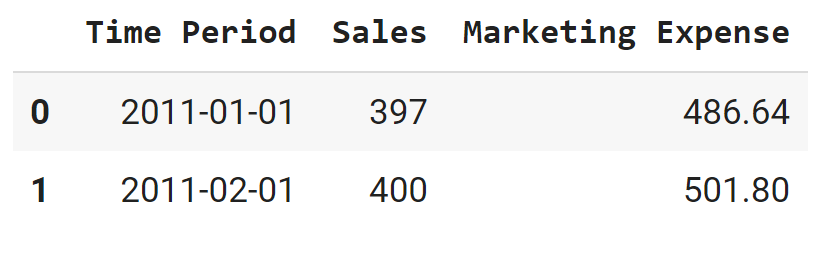Image by author: displaying first few rows from sales & marketing dataset.

Next, we visualize the data as seen below with the outcome attribute (i.e., sales in this particular case).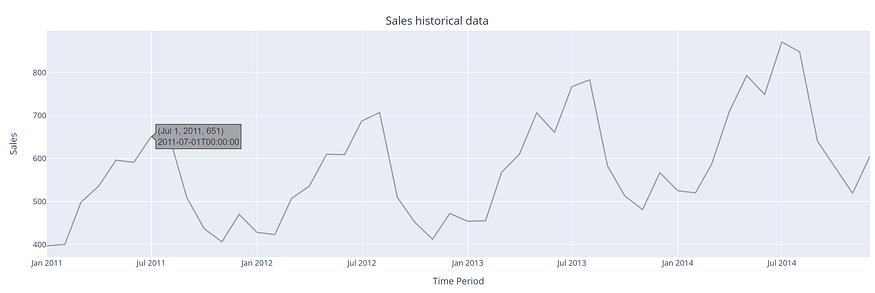Image by author: Interactive Graph Plot with respect to forecasting attribute.

Next, for feature engineering, we use the generate_date_time_features_month() function, followed by generating cyclic features from date using the generate_cyclic_features() method, and also generating other features relevant to the context of time-series data using the generate_other_related_features() method. Here, one can also use lagged observations as features. Further, one can also derive a bunch of several other useful features, as shown here.

### Training the forecast model

Once the feature engineering part is done, next we proceed with training the model using train() method either using the CPU/GPU resources as available, while providing the processed dataframe, outcome column, i.e., renamed as ‘value’ with the set/get methods, split ratio for train-validation-test set split, and all other previously pre-configured parameters as input to the method. The corresponding training results are shown below. We also plot the predictions made by the model against the actual values, as seen in the graph plot below. The results depicted here are just based on a few epochs without any hyper-parameter tuning done. One can certainly fine-tune the model further to improve the performance. We also evaluate the model with widely used evaluation metrics and plot them for side-by-side comparison. The interactive forecast plots are also plotted for the user-provided time window. The forecast model results are also outputted to disk as flat CSV files as well as interactive visualization plots can be saved to disk at ease.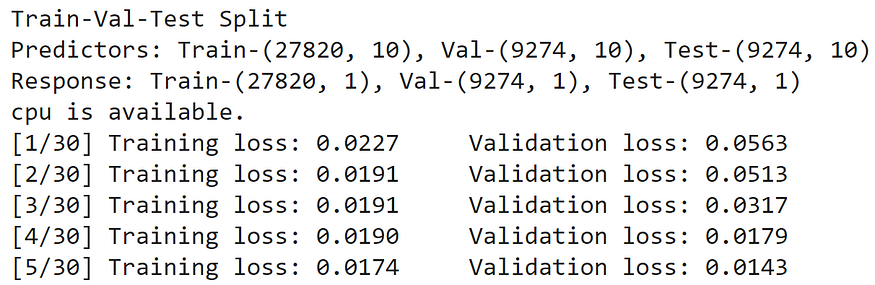Image by author: Displaying Train-Val-Test Split with available computational resource with training/val losses.

### Results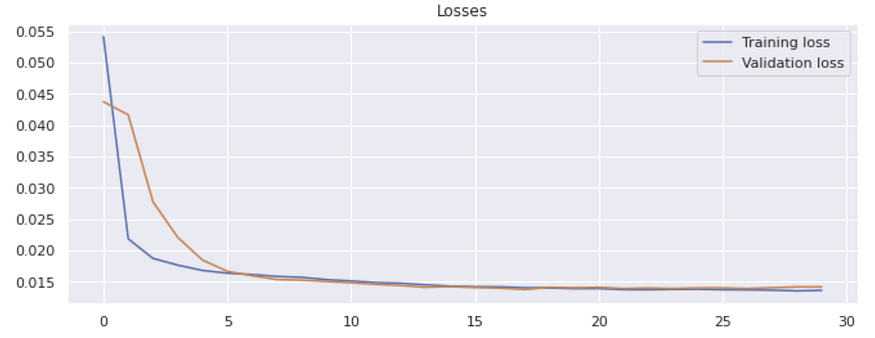Image by author: Model training-validation loss graph plot.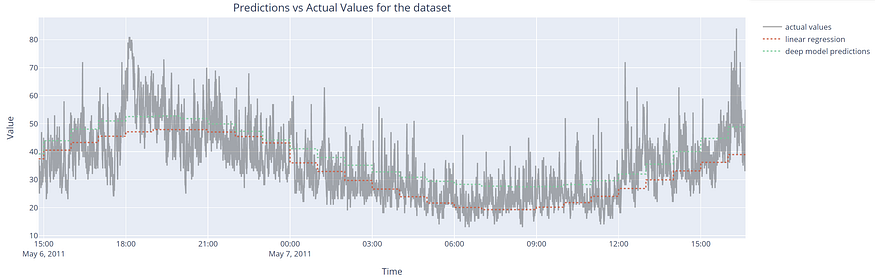Image by author: Interactive visualization plot displaying the predictions made by a linear model, and deep model.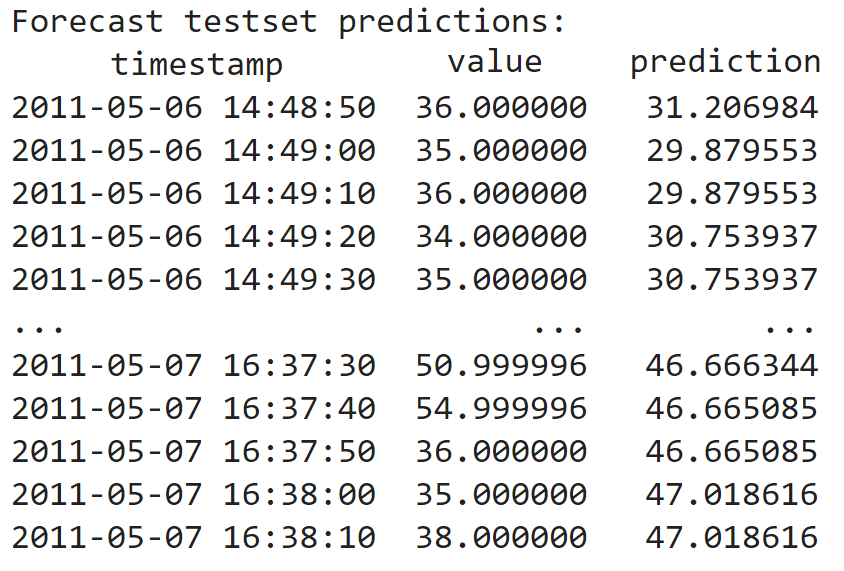Image by author: Forecast model validation for CPU utilization outcome of Alibaba cloud virtual machine’s dataset.

For a simple, intuitive explanation of the widely used regression metrics, check out this blog here. Further, one can also check out this library “regressormetricgraphplot” that can be used to easily compute several widely used regression evaluation metrics and plot them at ease with single line code for the different widely used machine learning algorithms, and also make a side-by-side comparison at a glance.

Let’s quickly glimpse through ‘SMAPE’, one of the estimated metrics among several other estimated ones by default such as r, MAE, RMSE, MAPE, RMSRE, variance score, etc. The symmetric mean absolute percentage error (SMAPE) is simply an accuracy measure based on percentage (or relative) errors. The percentage errors, in general, are simple to understand and interpret. In layman terms, it gives the estimate of the infiniteness of the forecasts that are higher than the actuals.

The SMAPE is calculated as shown in the python snippet syntax below.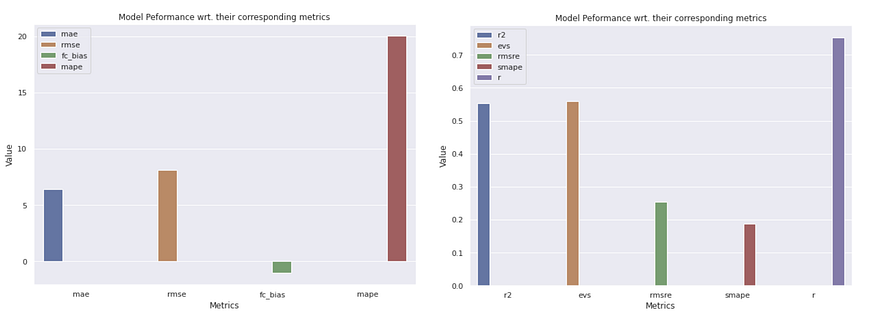Image by author: Model performance bar plot with widely used key evaluation metrics.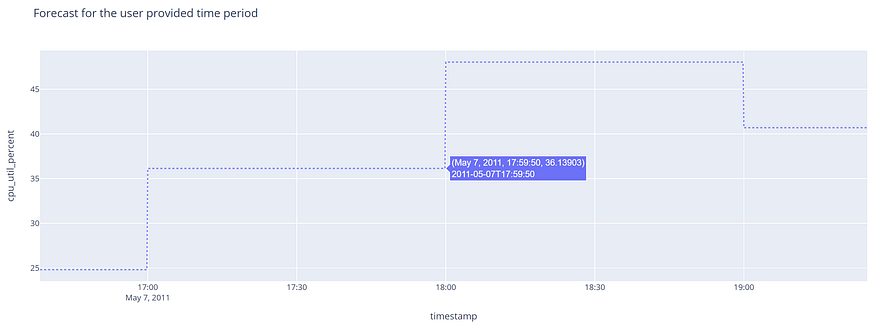Image by author: Interactive visualization plot of the forecasts.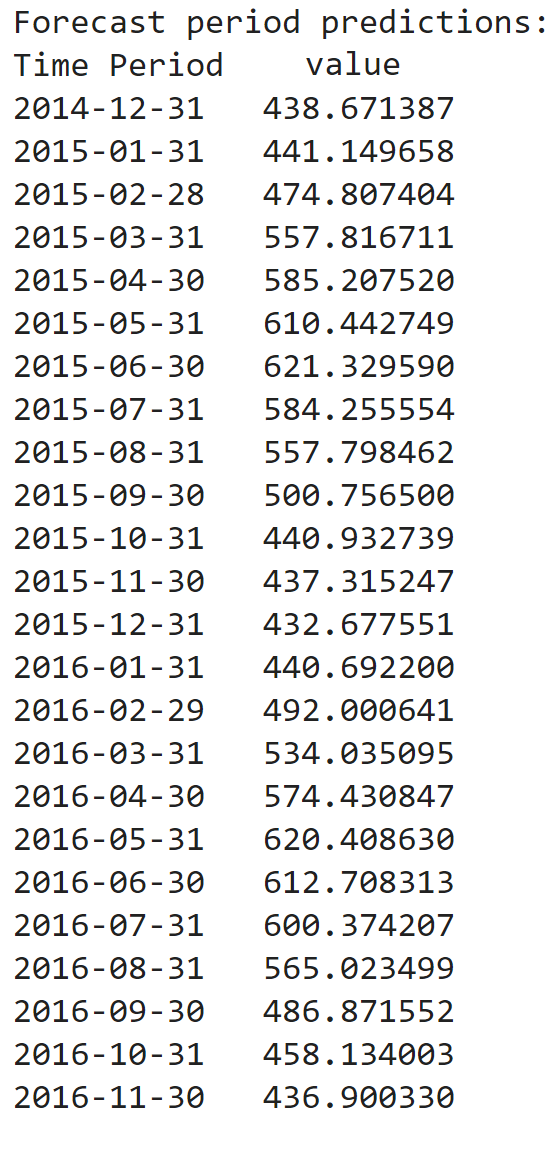Image by author: Forecast model inferences made for sales outcome.

### Bias Estimation & Model Explainability

Forecast Bias can be described as a tendency to either over-forecast (i.e., the forecast is more than the actual), or under-forecast (i.e., the forecast is less than the actual), leading to a forecasting error. The forecast bias can be estimated using the formulae as shown below.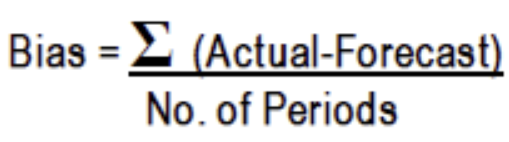Image by author: Forecast Bias mathematical formula.

The algorithm computes the forecast bias as shown with the python snippet syntax below outputting the estimated bias as seen in image below, and also estimating several key metrics & plotting them as bar plot.

fc_bias’ : (np.sum(np.array(df.value) — np.array(df.prediction)) * 1.0/len(df.value))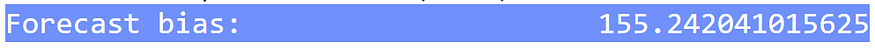Image by author: Forecast Bias Estimation.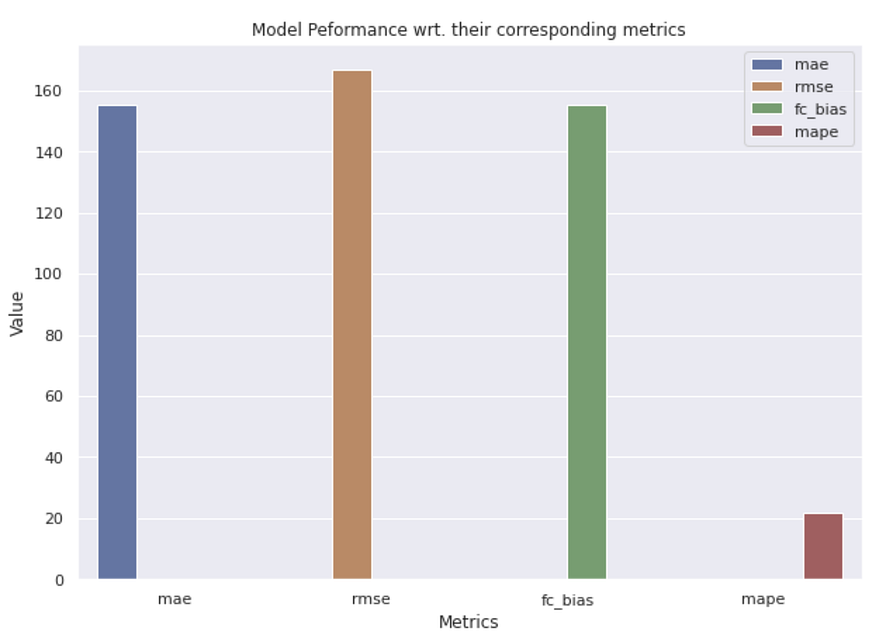Image by author: Bar plot with forecast model evaluation metrics.

Finally, we proceed with getting the interpretations of the model using the explainable_forecast() method for the required specific forecast input sample. We also plot the shapely values to understand the feature value contribution towards the particularly made model inference.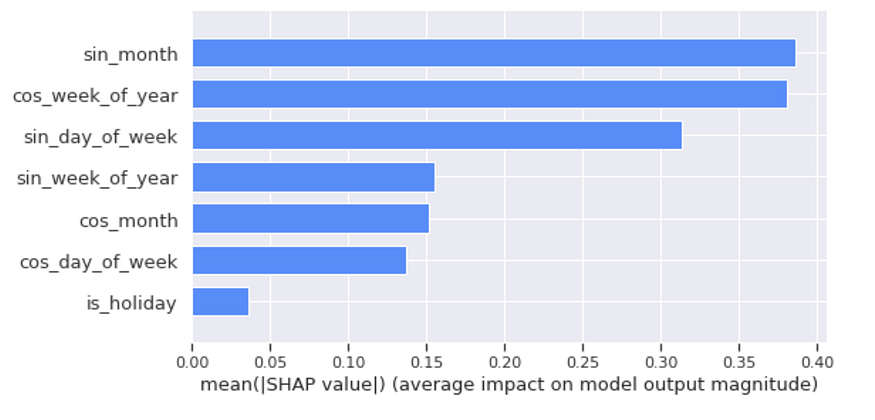Image by author: Model Explainability based on the derived features for forecasted data point.

The above graph plot is the result of a forecasted data sample. It can be seen that the features that contributed significantly to the corresponding model inference towards the outcome column based on the derived datetime, cyclic, and other generated features are especially the reference to a particular month, the significance of a particular week of the year, ones that imply the importance with respect to a corresponding day of a week, whether a holiday, in particular, was of any significant or not, etc.

Note: This package is still by large a work in progress, so always open to your comments and things that you feel should also be included. Also, if you want to be a contributor, you are always most welcome. Please get in touch if you are interested in being a contributor in any capacity.

The RNN/LSTM/GRU/BiRNN/BiGRU are already part of the initial version release, while the Spiking Neural Network, Graph Neural Network, Transformers, GAN, BiGAN, DeepCNN, DGCNN, LSTMAE, and many more are under testing and will be added soon once the testing is completed.

### Conclusion

We have seen how intuitively deep learning-based forecasting models can be built at ease with just a few lines of code with the user-specific custom inputs without the need of actually designing the deep network architectures all from scratch. We walked through two different use cases of forecasting scenarios.

DeepXF helps in designing complex forecasting and nowcasting models with built-in utility for time series data. One can automatically build interpretable deep forecasting and nowcasting models at ease with this simple, easy-to-use, and low-code solution. With this library, one can easily do Exploratory Data Analysis with services like data profiling, filtering outliers, creating univariate/multivariate plots, plotly interactive plots, rolling window plots, detecting peaks, etc. and also easy data preprocessing for their time-series data with services like finding missing, imputing missing, date-time extraction, single timestamp generation, removing unwanted features, etc. One can also get quick descriptive statistics for the provided time-series data. It also provides the possibility of feature engineering with services like generating time lags, date-time features, one-hot encoding, date-time cyclic features, etc. Also, it caters to the signal data where one can quickly find similarities between two homogeneous time-series signal inputs. It provides a facility to remove noise from time-series signal inputs, etc.

It would be a promising direction to detect spikes in given such temporal space settings and build a forecast model with the Hebbian learning rule. If you are keen, then certainly do check out my other work in context to building predictive models with Spiking Neural Networks from here.

Here’s the complete Google Colab Notebook link for both usecase-1 and usecase-2 discussed in this post.

Original. Reposted with permission.